# QCM on quadratic forms, equations and inequalities

To each question, there is one, and only one correct answer. Each correct answer has 2 points, while a wrong answer takes 0.5. The lack of response does not report or render any point.
Note: Even if questions are presented as a QCM where only a single "click" is required to answer a question, it can be useful (necessary and unavoidable in my opinion) to bring a sheet of paper and take the time to write down calculations before "clicking".

See also: Mathematic course on quadratic an polynomial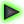Q1: Solutions of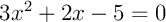are:
 not existing x=1 x=1 et x=-5/3 x=-1 et x=5/3

Q2: Solutions of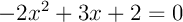are:
 No solution x=-4 et x=1 x=-4 et x=1 x=2 et x=-1/2

Q3: Solutions ofare:
 No solution x=-6 et x=3 x=-12 et x=6 x=-24 et x=12

Q4: Solutions of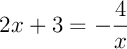are:
 No solution x=-1 et x=3 x=-12 et x=6 x=-24 et x=12

Q5: Solutions ofare:
 Aucune solution x=0 x=0 et x=2 et x=-5 x=2 et x=-5

Q6: Solutions of the inequalityare:
 No solution -3< x <4 x<-3 ou x>4 x<-3

Q7: The solutions of the inequalityare:
 No solution x <-6 ou x >3 -6< x <-5 ou 2< x < 3 x<-3

Q8: Solutions of the inequality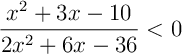are:
 no solution x <-6 ou x >3 -6< x <-5 ou 2< x < 3 x<-3